Everyone is welcome here --- except those who have borrowed books from me for and have not returned them yet!

# Introduction to mixed-effects modelling

Posted on mai 29, 2017 in stats

# Introduction to mixed-effects modelling

Christophe Pallier
2017-05-29

### Plan

• Some generalities about statistical inference
• Classic regression and its implementation in R
• Random effects and mixed-effects models
• Examples of mixed-effects analyses with R

### Meaning of "X has an effect on Y" mean

What does it mean, from a statistical point of view, to say that the variable $$X$$ has an effect on the variable $$Y$$?

$$Y$$ is influenced by $$X$$ if the *distribution of $$Y$$ change when $$X$$ takes different values.

### Example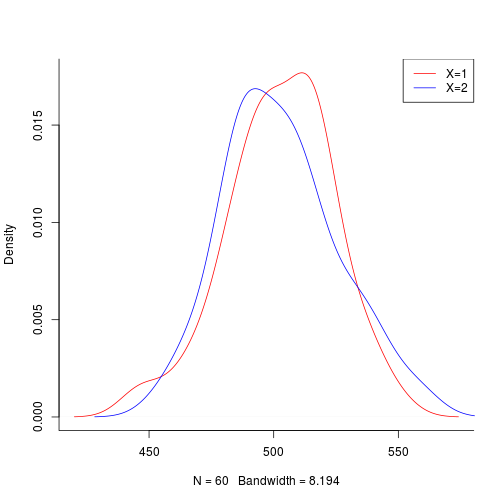As the two distributions differ, we can state that “$$X$$ has an effect on $$Y$$”“ (not implying causality).

Remark: One is often only interested in whether the means of the distributions differ, e.g.: $E(Y|X=1) \stackrel{?}{=} E(Y|X=2)$

### Sampling

In real life, we rarely have access to the data of the whole population but only to random samples, that is, to a finite set of observations $$(y_i)$$.

The distributions of the samples can (and often will) differ from one another because of sampling variability, that is, the fact that different individuals compose each sample.

For example, the mean heights of two groups of 20 boys will not be exactly the same.

### Inference

To make statements about a population property from observed samples, we need statistical inference.

1. hypothesis testing (e.g. does the mean of $$Y$$ (e.g. height) varies with $$X$$ (e.g. nationality)?)
2. estimation (e.g. what is a confidence interval for the average height of French people?)
3. prediction (e.g. what is the most probable height of a 18 year old German boy?)

### Classical Simple Regression

• One predictor variable $$X$$
• One dependent variable $$Y$$ (continuous).

The question of interest is whether $$E(Y|X)$$ is constant or not:

$\cal{H}_0: \quad \rm E(Y|X=x) = \beta, \quad x \ \mbox{varying}$

One alternative hypothesis is that the average of $$Y$$ depends linearily on $$X$$ :

$\cal{H}_{lin}: \quad \rm E(Y|X=x) = \alpha x + \beta$

### Graphical Representation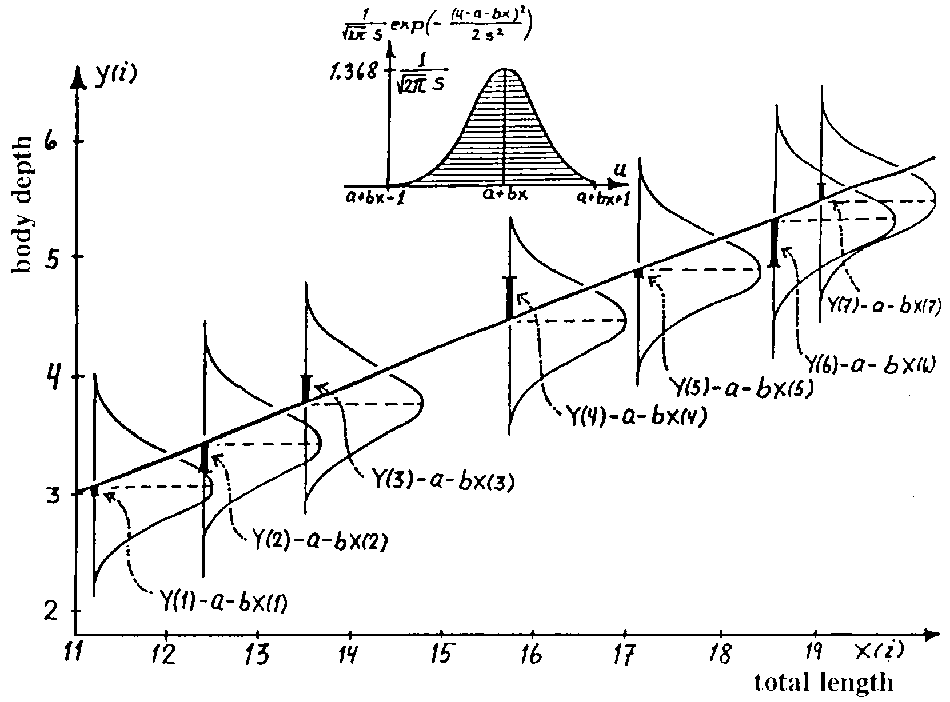### Example

Effect of age on tot, a biomarker of kidney functioning.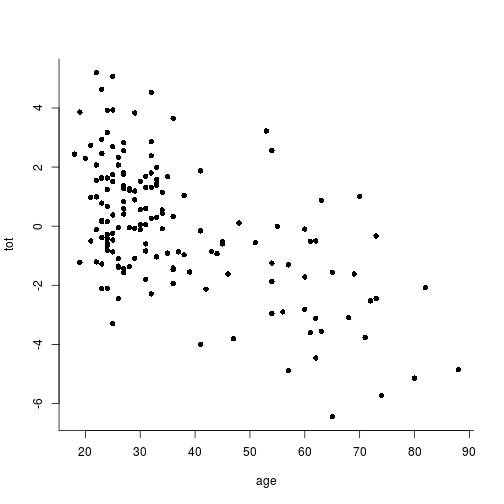### Ordinary Least Squares solution to Regression

The OLS method searches among all linear models one that minimizes the Euclidian distance between the model's predictions and the data ($$\sum (y_i - \hat{y}_i)^2$$).

In R, this is accomplished by the fit function:

x = age
y = tot
fit = lm(y ~ x)
coef(fit)

(Intercept)           x
2.86002680 -0.07858842


These coefficients estimate the population parameters $$\beta$$ and $$\alpha$$.

### Prediction

The equation $$\alpha.x+\beta$$ can be used to compute the expected value of $$Y$$ for a given value $$x$$ of $$X$$

plot(y ~ x, bty='l', pch=16)
abline(fit, col=2, lwd=2)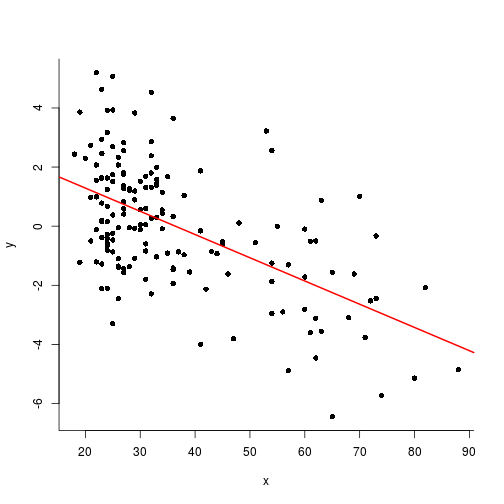### Hyothesis testing in simple regression

To draw any inference from these coefficients to the population parameters, for example to know their precision, you need an additional hypothesis.

The simplest one is the the errors terms are statistically independent and each is distributed following a normal distribution with a standard deviation $$\sigma$$ :

$y_i = \alpha . x_i + \beta + \epsilon_i , \quad \epsilon \sim \cal{N}(0,\sigma^2)$

### Testing the hypothesis of a non-null slope

The p-value for the hypothesis that $$X$$ has no (linear) effect on $$Y$$ can be obtained calling the function anova with the linear model object as a parameter.

anova(fit)

Analysis of Variance Table

Response: y
Df Sum Sq Mean Sq F value    Pr(>F)
x           1 244.29 244.288  75.312 5.182e-15 ***
Residuals 155 502.77   3.244
---
Signif. codes:  0 '***' 0.001 '**' 0.01 '*' 0.05 '.' 0.1 ' ' 1


### Estimates and standard errors of coefficients

They can be displayed with the function summary, allowing to construct confidence intervals for them:

summary(fit)


Call:
lm(formula = y ~ x)

Residuals:
Min      1Q  Median      3Q     Max
-4.2018 -1.3451  0.0765  1.0719  4.5252

Coefficients:
Estimate Std. Error t value Pr(>|t|)
(Intercept)  2.860027   0.359565   7.954 3.53e-13 ***
x           -0.078588   0.009056  -8.678 5.18e-15 ***
---
Signif. codes:  0 '***' 0.001 '**' 0.01 '*' 0.05 '.' 0.1 ' ' 1

Residual standard error: 1.801 on 155 degrees of freedom
Multiple R-squared:  0.327, Adjusted R-squared:  0.3227
F-statistic: 75.31 on 1 and 155 DF,  p-value: 5.182e-15


### Remark 1: X can be binary

The regression regression of $$Y$$ on $$X$$ is strictly equivalent to a Student T test.

x = rep(1:2, 20)
y = x + rnorm(40)

m1 <- lm(y ~ x)
plot(y ~ x, pch=16, xlim=c(.5, 2.5))
abline(m1)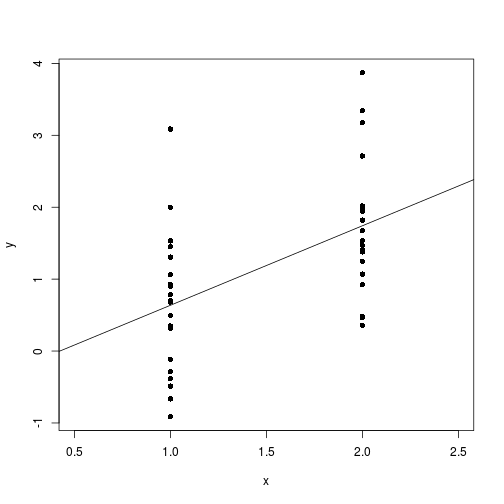### Case of binary X

summary(m1)


Call:
lm(formula = y ~ x)

Residuals:
Min       1Q   Median       3Q      Max
-1.55111 -0.69204 -0.01078  0.32311  2.45041

Coefficients:
Estimate Std. Error t value Pr(>|t|)
(Intercept)  -0.4690     0.4822  -0.973 0.336914
x             1.1057     0.3050   3.626 0.000842 ***
---
Signif. codes:  0 '***' 0.001 '**' 0.01 '*' 0.05 '.' 0.1 ' ' 1

Residual standard error: 0.9644 on 38 degrees of freedom
Multiple R-squared:  0.257, Adjusted R-squared:  0.2375
F-statistic: 13.15 on 1 and 38 DF,  p-value: 0.0008421

t.test(y ~ x, var.equal=T)


Two Sample t-test

data:  y by x
t = -3.6258, df = 38, p-value = 0.0008421
alternative hypothesis: true difference in means is not equal to 0
95 percent confidence interval:
-1.7230634 -0.4883563
sample estimates:
mean in group 1 mean in group 2
0.6367533       1.7424631


### Remark 2: testing for non linear effect of X

x = age
y = tot
m1 <- lm(y ~ x)
summary(m2 <- lm(y ~ poly(x, 2))) # in '*linear model*', '*linear*' stands for a the relationship between the parameters and Y, not the predictors and Y


Call:
lm(formula = y ~ poly(x, 2))

Residuals:
Min      1Q  Median      3Q     Max
-4.2024 -1.3492  0.0449  1.0755  4.4781

Coefficients:
Estimate Std. Error t value Pr(>|t|)
(Intercept) -1.911e-04  1.442e-01  -0.001    0.999
poly(x, 2)1 -1.563e+01  1.806e+00  -8.652 6.26e-15 ***
poly(x, 2)2 -4.758e-01  1.806e+00  -0.263    0.793
---
Signif. codes:  0 '***' 0.001 '**' 0.01 '*' 0.05 '.' 0.1 ' ' 1

Residual standard error: 1.806 on 154 degrees of freedom
Multiple R-squared:  0.3273,    Adjusted R-squared:  0.3186
F-statistic: 37.46 on 2 and 154 DF,  p-value: 5.522e-14


### Remark 2: testing for non linear effect of X

plot(x, y)
lines(x, fitted(m1), col=2) # linear
lines(x, fitted(m2), col=3) # polynomial deg 2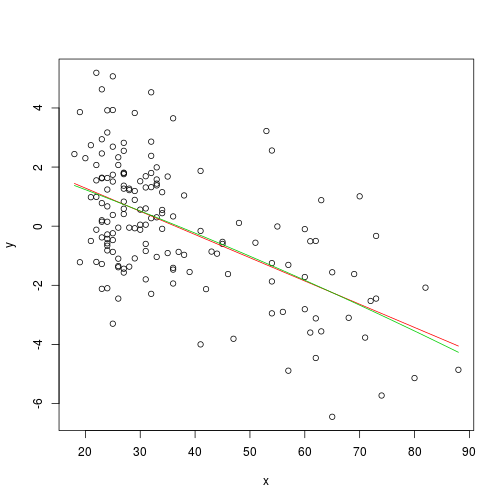### Remark 3: Non-parametric analyses as an alternative

plot(y ~ x, bty='l', pch=16)
lines(lowess(x, y), col='blue', lwd=2)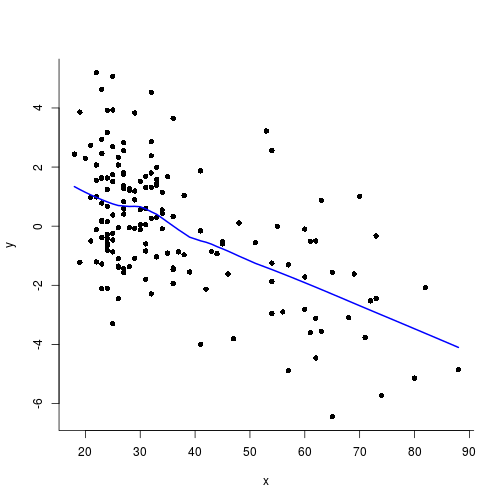### Remark 4: nicer plot with ggplot2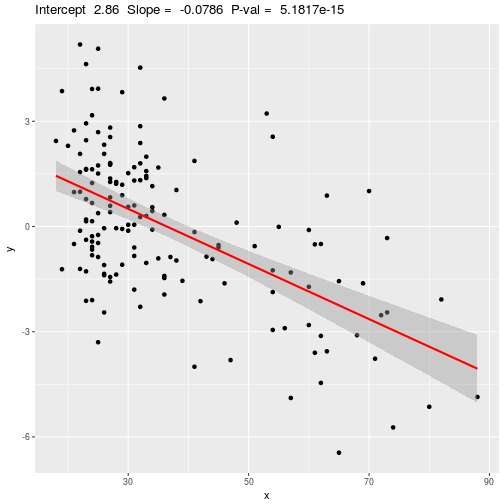### Multiple regression

Mutliple regression is a simple extension of simple regression: instead of considering only one predictor variable '$$X$$', several are taken into account '$$X_1, X_2, ... X_p$$'. The linear model then becomes:

$y_i = \beta_1.x_{1i}+ \beta_2.x_{2i} + ... + \beta_p.x_{pi} + \epsilon_i$

### Interpretation of coefficients

$$\beta_i$$ represents the amount by which $$Y$$ is modified when $$X_i$$ increases by 1 while the other $$X_{j \neq i}$$ remain constant (all other things equal).

Remark: The interpretation of $$\beta_i$$ can actually become tricky when the $$\X_i$$ are highly correlated (see variance inflation factor, VIF).

For categorical variables (factors), the interpretation of the betas depends on the coding contrast used: reference coding ( contr.treament) or deviation coding (contr.sum)

### Recommended readings for linear modelling

• John Fox (1997) Applied Regression Analysis, Linear Models, and Related Methods, Sage publications.
• Julian Faraway (2005) Linear Models with R, Chapman & Hall.
• Scott E. Maxwell and Harold D. Delaney (2000) Designing experiments and analyzing data: a model comparison perspective, Erlbaum.
• Frank Harrell (2001) Regression Modeling Strategies

### An issue with repeated measurements

Consider an experiment in which reaction times are recorded in two experimental conditions in human participants. Moreover let us suppose that each participant produced many data points in each condition (“repeated measures”).

 subj    cond   RT
s01      c1    520
s01      c1    497
s01      c1    489
...
s01      c2    530
s01      c2    513
...
s02      c1    632
s02      c1    567


### An issue with repeated measurements

Newcomers to Psychology are often surprised when they are told that:

RTs within subject and condition must be averaged before running the Anova (or t-test).

• Why it is not possible to perform the statistical analyses on the raw data set?
• What is the purpose of having several trials per subject if we average in the end?

### Justifications for averaging over repeated measurements

• the within-subject within-condition RTs are not independent (for example RTs may be autocorrelated), violating the assumption of statistical independence.

• Even if within subject data are independent, the mere fact that the the data is clustered by participants yields a lack of independence (see Intraclass Correlation Coefficient)

• the unit of observation over which we want to perform inference is generally the population of humans, not the population of trials.

One wants to compare the observed effect size to the variability between participants, not the trial-to-trial variability (A similar issue exists in fMRI data analyses: see first level/fixed effect vs. second level/random effects analyses. Unless you are interested in aprecise individual participant; think about single case studies in neuropsychology or psychophysics).

### One issue with averaging

This experiment actually has three sources of variations:

• the factor condition
• the factor subject
• the factor trial

Averaging over trials entails confuses the trial-to-trial variability and the between subjects variability.

(Remark: The reason to increase the number of within subject measurements is to decrease the weight of the trial-to-trial variabililty).

What are fixed and random factors?

### Fixed and Random factors

• Fixed factor: we are interested in differences between the levels of the factor.

• Random factor: the levels would vary in replications of the experiment. The effect for a given level is not of interest, but we may be interest in the variance.

Relations between factors: two factors can be crossed, nested or neither.

Knowing the relations between factors and their types allows one to compute the Expected Mean Squares in an ANOVA, and select the appropriate error term to assess an effect.

(See, e.g. Abdi, Edelman, Valentin, Dowling (2009) Experimental Design and Analysis for Psychology, OUP.)

### The score model

One reasonable model for the score of the kth trial of the jth subject in condition i is the following: $Y_{ijk} = \mu + \beta_i + s_j + \epsilon_{ijk}$ Where:

• $$\beta_i$$ corresponds to the effect of the ith condition (measured as a distance from the global mean $$\mu$$)
• $$s_j$$ corresponds to the effects of the jth subject
• $$s_j \sim {\cal N}(0, \sigma_s^2)$$
• $$\epsilon_{ijk}$$ is the effect of trial 'ijk'
• $$\epsilon_{ijk} \sim {\cal N}(0, \sigma_\epsilon^2)$$

### Mixed-effects

The problem is that most data analysis softwares used to allow only a single random factor. And statistics textbooks for psychology only explained the procedures to analyse design with a single random factor even though most experiments have two or more.

“Mixed-effects” procedures allows one to take into account the presence of several random factors, and estimate the various sources of variation. They have many advantages over classic ANOVA.

### Advantages of mixed-effect modelling over ANOVA and rANOVA

• better handling of missing data, between group unbalance, heteroscestadicity.
• avoid kludges like Greenhouse-Geisser correction for non-sphericity.
• They provide less biased estimate of individual effects (by shrinkage; see B. Efron & C. Morris (1977) Stein's Paradox in Statistics. Scientific American, pp. 119–127).
• allow more detailed modeling of effects as it is possible to incorporate trial level predictors in the model.
• encourage to better look at the data.

one can get more from one's data than with traditional ANOVA techniques.

### Drawback of mixed effect modelling

• relative complexity (need to really master multiple regression first)

• unnecessary in simple cases where the assumptions of ANOVA hold
• synthesizing the results of analysis can be more challenging (one can hope that, one day, the results section of scientific articles will be replaced by the full outputs of data analysis scripts).

### First example

Let us generate a dataset with 2 conditions and repeated measures within participants.

### First example

data1 <- data.frame(sub, cond, y)
str(data1)

'data.frame':   800 obs. of  3 variables:
$sub : Factor w/ 20 levels "1","2","3","4",..: 1 1 1 1 1 1 1 1 1 1 ...$ cond: Factor w/ 2 levels "1","2": 1 1 1 1 1 1 1 1 1 1 ...
$y : num -34.9 186.7 110.3 -58.5 140.4 ...  head(data1)   sub cond y 1 1 1 -34.86155 2 1 1 186.66035 3 1 1 110.26327 4 1 1 -58.45421 5 1 1 140.37307 6 1 1 72.39533  ### First example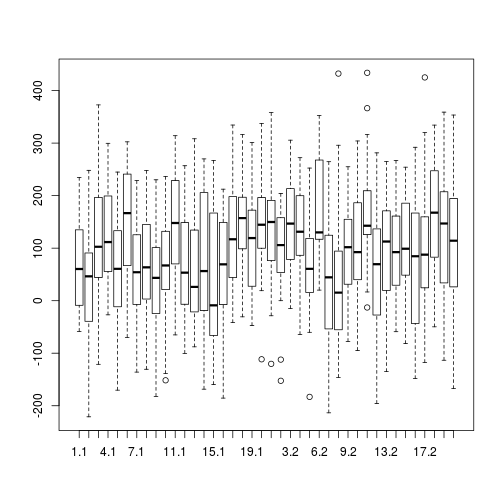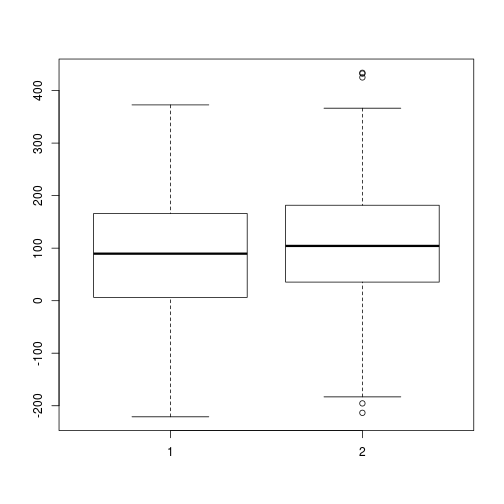### Classical Anova bysub <- aggregate(y ~ cond + sub, data=data1, mean) with(bysub, { print(tapply(y, cond, mean)); interaction.plot(cond, sub, y)})   1 2 88.45269 106.35811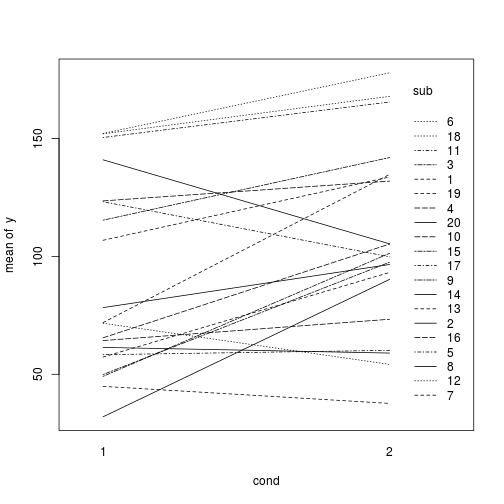### Classical anova summary(anov <- aov(y ~ cond + Error(sub/cond), data=bysub))   Error: sub Df Sum Sq Mean Sq F value Pr(>F) Residuals 19 53106 2795 Error: sub:cond Df Sum Sq Mean Sq F value Pr(>F) cond 1 3206 3206 8.683 0.00828 ** Residuals 19 7016 369 --- Signif. codes: 0 '***' 0.001 '**' 0.01 '*' 0.05 '.' 0.1 ' ' 1  ### Mixed-effects model require(lme4) require(lmerTest) lme1 <- lmer(y ~ cond + (1 | sub), data=data1) summary(lme1)  Linear mixed model fit by REML t-tests use Satterthwaite approximations to degrees of freedom [lmerMod] Formula: y ~ cond + (1 | sub) Data: data1 REML criterion at convergence: 9761.7 Scaled residuals: Min 1Q Median 3Q Max -2.7541 -0.6748 -0.0146 0.7129 3.3294 Random effects: Groups Name Variance Std.Dev. sub (Intercept) 1112 33.35 Residual 11406 106.80 Number of obs: 800, groups: sub, 20 Fixed effects: Estimate Std. Error df t value Pr(>|t|) (Intercept) 88.453 9.172 27.500 9.643 2.54e-10 *** cond2 17.905 7.552 779.800 2.371 0.018 * --- Signif. codes: 0 '***' 0.001 '**' 0.01 '*' 0.05 '.' 0.1 ' ' 1 Correlation of Fixed Effects: (Intr) cond2 -0.412  ### Estimated subject's' effects $sub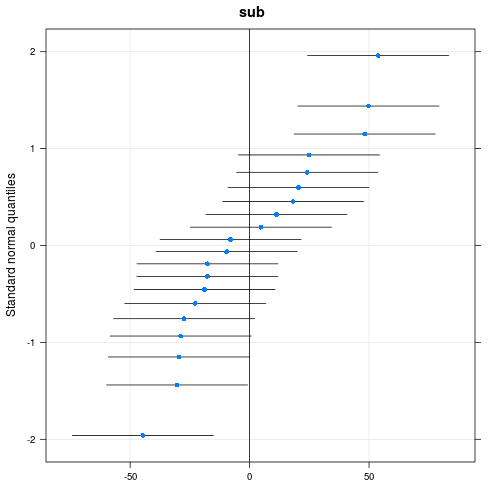We assumed that the effect of condition was the same across all subjects, but we can fit a more flexible model allowing for a random effect of cond within subject

### Fitting a more flexible model

lme2 <- lmer(y ~ cond + (1 + cond | sub), data=data1)
summary(lme2)

Linear mixed model fit by REML t-tests use Satterthwaite approximations
to degrees of freedom [lmerMod]
Formula: y ~ cond + (1 + cond | sub)
Data: data1

REML criterion at convergence: 9761.7

Scaled residuals:
Min      1Q  Median      3Q     Max
-2.7542 -0.6746 -0.0121  0.7139  3.3247

Random effects:
Groups   Name        Variance  Std.Dev. Corr
sub      (Intercept) 1.143e+03  33.8018
cond2       8.068e-01   0.8982 -1.00
Residual             1.141e+04 106.7993
Number of obs: 800, groups:  sub, 20

Fixed effects:
Estimate Std. Error      df t value Pr(>|t|)
(Intercept)   88.453      9.254  20.000   9.558 6.85e-09 ***
cond2         17.905      7.555 732.200   2.370    0.018 *
---
Signif. codes:  0 '***' 0.001 '**' 0.01 '*' 0.05 '.' 0.1 ' ' 1

Correlation of Fixed Effects:
(Intr)
cond2 -0.430


Let us compare both models:

anova(lme2, lme1)

Data: data1
Models:
..1: y ~ cond + (1 | sub)
object: y ~ cond + (1 + cond | sub)
Df    AIC    BIC  logLik deviance  Chisq Chi Df Pr(>Chisq)
..1     4 9781.6 9800.3 -4886.8   9773.6
object  6 9785.6 9813.7 -4886.8   9773.6 0.0115      2     0.9943


This allows us to test whether there is significant variance in the effect of condition across subjects.

### Second example: simple regressions within subjects

To test if a continuous predictor $$X$$ has an effect on a continuous variable $$Y$$, the classic linear regression framework assumes that measurements are independent.

x <- rnorm(100)
y <- 5 + x + rnorm(100)
plot(y ~ x, pch=16)
summary(lm1 <- lm(y ~ x))


Call:
lm(formula = y ~ x)

Residuals:
Min       1Q   Median       3Q      Max
-3.04035 -0.52200 -0.02043  0.72914  1.91953

Coefficients:
Estimate Std. Error t value Pr(>|t|)
(Intercept)  4.88012    0.09464   51.57   <2e-16 ***
x            1.03755    0.09589   10.82   <2e-16 ***
---
Signif. codes:  0 '***' 0.001 '**' 0.01 '*' 0.05 '.' 0.1 ' ' 1

Residual standard error: 0.944 on 98 degrees of freedom
Multiple R-squared:  0.5443,    Adjusted R-squared:  0.5397
F-statistic: 117.1 on 1 and 98 DF,  p-value: < 2.2e-16

abline(lm1)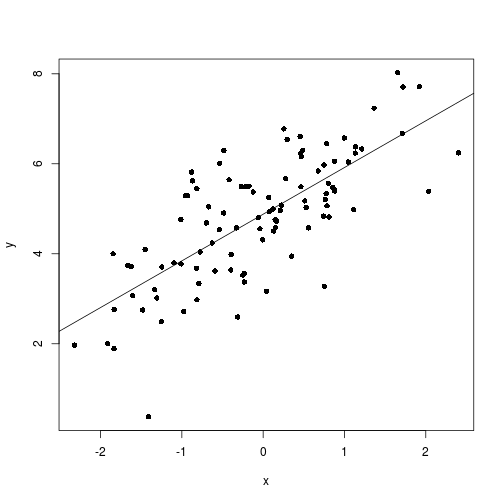### Clustered measurements

Let us suppose that the measures are “clustered”, that is, come from different participants. The independence assumption is violated.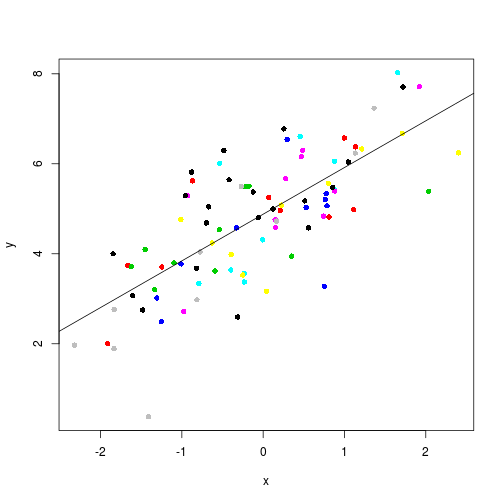### Classical approaches: group as a fixed factor

summary(lm(y ~ x + g))


Call:
lm(formula = y ~ x + g)

Residuals:
Min       1Q   Median       3Q      Max
-2.62106 -0.63273  0.07911  0.58336  1.80567

Coefficients:
Estimate Std. Error t value Pr(>|t|)
(Intercept)  5.22779    0.29648  17.633   <2e-16 ***
x            1.02948    0.10095  10.198   <2e-16 ***
g2           0.01246    0.42167   0.030   0.9765
g3          -0.28507    0.41968  -0.679   0.4987
g4          -0.42151    0.42364  -0.995   0.3225
g5          -0.79667    0.41880  -1.902   0.0604 .
g6          -0.36249    0.41832  -0.867   0.3885
g7          -0.21226    0.41848  -0.507   0.6133
g8          -0.69352    0.41885  -1.656   0.1013
g9          -0.77835    0.42721  -1.822   0.0718 .
g10          0.05507    0.42028   0.131   0.8960
---
Signif. codes:  0 '***' 0.001 '**' 0.01 '*' 0.05 '.' 0.1 ' ' 1

Residual standard error: 0.9354 on 89 degrees of freedom
Multiple R-squared:  0.5937,    Adjusted R-squared:  0.5481
F-statistic: 13.01 on 10 and 89 DF,  p-value: 1.05e-13


### Classical approach II: random regressions

Another approach would be to compute the regression lines within each subgroups and see if the slopes are significantly different from 0.

require(lme4)
summary(ll <- lmList(y ~ x | g, data=data.frame(g, x, y), pool=FALSE))

Call:
Model: y ~ x | NULL
Data: data.frame(g, x, y)

Coefficients:
(Intercept)
Estimate Std. Error  t value     Pr(>|t|)
1  5.279415  0.3127493 16.88066 4.317584e-28
2  5.148072  0.3222263 15.97657 1.304357e-26
3  4.926271  0.2932141 16.80093 5.807291e-28
4  4.561703  0.3194453 14.28007 1.034148e-23
5  4.431121  0.2911254 15.22066 2.440710e-25
6  4.757161  0.2985573 15.93383 1.536352e-26
7  4.966494  0.3131747 15.85854 2.050923e-26
8  4.595111  0.3130507 14.67849 2.086530e-24
9  4.784621  0.3326146 14.38488 6.774336e-24
10 5.275362  0.2963988 17.79819 1.508026e-29
x
Estimate Std. Error  t value     Pr(>|t|)
1  0.7709056  0.5723844 1.346832 1.818374e-01
2  0.7469003  0.4233653 1.764198 8.151595e-02
3  0.9081946  0.2575459 3.526340 7.007535e-04
4  0.5024107  0.2833711 1.772978 8.003867e-02
5  0.9475340  0.3446890 2.748953 7.387759e-03
6  1.6784043  0.3972504 4.225054 6.284580e-05
7  1.1840832  0.3638882 3.253976 1.668477e-03
8  0.8814299  0.2800796 3.147069 2.317080e-03
9  1.5374619  0.2438057 6.306095 1.474008e-08
10 0.9924809  0.2746013 3.614262 5.247836e-04

Residual standard error: 0.9206192 on 80 degrees of freedom

t.test(coef(ll)[,2])


One Sample t-test

data:  coef(ll)[, 2]
t = 8.9013, df = 9, p-value = 9.343e-06
alternative hypothesis: true mean is not equal to 0
95 percent confidence interval:
0.7570356 1.2729255
sample estimates:
mean of x
1.014981


### More principled approach: fit a multilevel regression

summary(lmer(y ~ x + (1 + x | g)))

Linear mixed model fit by REML t-tests use Satterthwaite approximations
to degrees of freedom [lmerMod]
Formula: y ~ x + (1 + x | g)

REML criterion at convergence: 273.8

Scaled residuals:
Min      1Q  Median      3Q     Max
-2.8193 -0.5823 -0.0392  0.7140  1.9780

Random effects:
Groups   Name        Variance Std.Dev. Corr
g        (Intercept) 0.006856 0.0828
x           0.053852 0.2321   -0.68
Residual             0.829530 0.9108
Number of obs: 100, groups:  g, 10

Fixed effects:
Estimate Std. Error      df t value Pr(>|t|)
(Intercept)  4.88407    0.09661 9.02700   50.56 2.18e-12 ***
x            1.01993    0.12170 9.67900    8.38 9.64e-06 ***
---
Signif. codes:  0 '***' 0.001 '**' 0.01 '*' 0.05 '.' 0.1 ' ' 1

Correlation of Fixed Effects:
(Intr)
x -0.085


### Price of houses as a function of latitude (Galwey; 2006)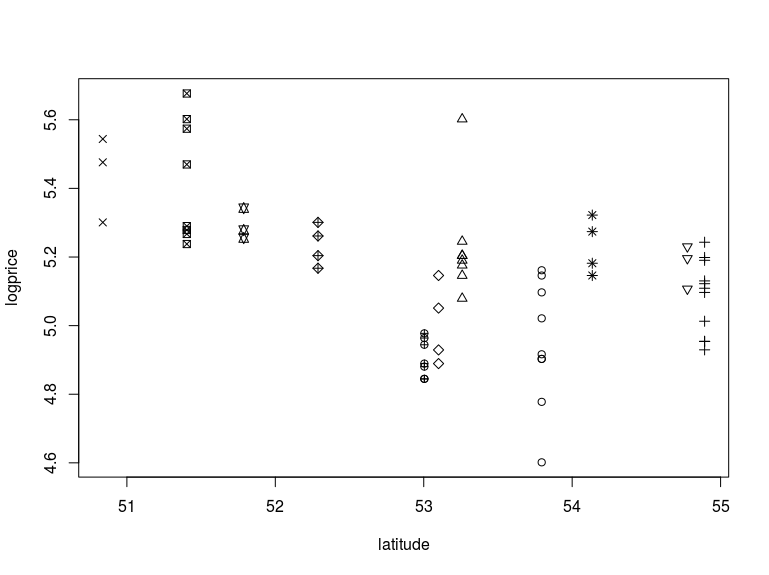### Models with several random factors are not a novelty !

• Clark, H. H. (1973) “The Language-as-Fixed-Effect Fallacy: A Critique of Language Statistics in Psychological Research.” Journal of Verbal Learning and Verbal Behavior 12: 335–59.

Clark remarked that most psycholinguistics experiments have (at least) two important random factors: subjects and items (words, sentences, …).

### Expected Mean Squares

Expected Mean squares for SxW<T> design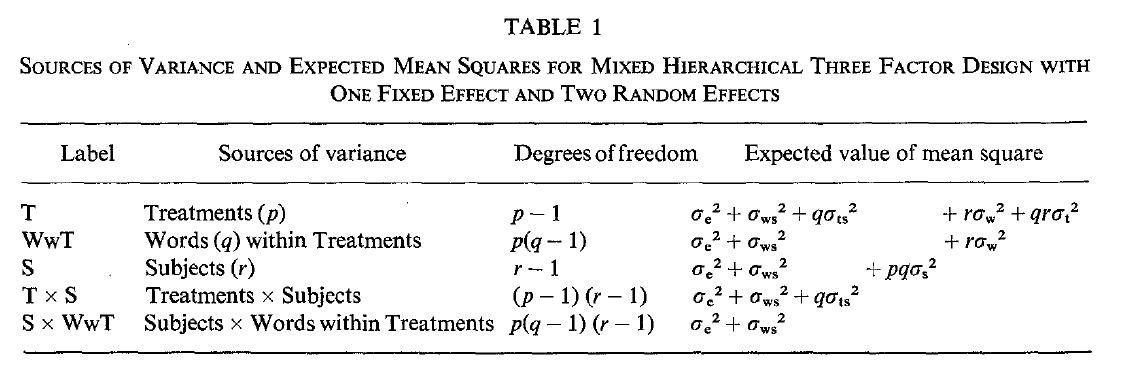Notice that there is no simple ratio between sources of variance to test the hypothesis that $$\sigma_t > 0$$.

For example, EMS$$_T$$ exceeeds EMS$$_{T{\rm x}S}$$ by $$\sigma_w^2 + qr\sigma_t^2$$.

### MinF'

Clark proposed a minimal bound, MinF' for a quasi-F ratio, that can be computed from $$F_1$$ (by subject) and $$F_2$$ (by item) analyses.

But using a mixed effect procedure, it is possible to avoid this cumbersome approach and to be more sensitive to detect an effect of Treatment (see Baayen (2006) Analysing Linguistics Data)

### Example of a lexical decision experiment

Dataset lexdec described in Baayen (2006) Analyzing Linguistic Data and provided in the languageR package.

require(languageR)
data(lexdec)
help(lexdec)
str(lexdec)

'data.frame':   1659 obs. of  28 variables:
$Subject : Factor w/ 21 levels "A1","A2","A3",..: 1 1 1 1 1 1 1 1 1 1 ...$ RT            : num  6.34 6.31 6.35 6.19 6.03 ...
$Trial : int 23 27 29 30 32 33 34 38 41 42 ...$ Sex           : Factor w/ 2 levels "F","M": 1 1 1 1 1 1 1 1 1 1 ...
$NativeLanguage: Factor w/ 2 levels "English","Other": 1 1 1 1 1 1 1 1 1 1 ...$ Correct       : Factor w/ 2 levels "correct","incorrect": 1 1 1 1 1 1 1 1 1 1 ...
$PrevType : Factor w/ 2 levels "nonword","word": 2 1 1 2 1 2 2 1 1 2 ...$ PrevCorrect   : Factor w/ 2 levels "correct","incorrect": 1 1 1 1 1 1 1 1 1 1 ...
$Word : Factor w/ 79 levels "almond","ant",..: 55 47 20 58 25 12 71 69 62 1 ...$ Frequency     : num  4.86 4.61 5 4.73 7.67 ...
$FamilySize : num 1.386 1.099 0.693 0 3.135 ...$ SynsetCount   : num  0.693 1.946 1.609 1.099 2.079 ...
$Length : int 3 4 6 4 3 10 10 8 6 6 ...$ Class         : Factor w/ 2 levels "animal","plant": 1 1 2 2 1 2 2 1 2 2 ...
$FreqSingular : int 54 69 83 44 1233 26 50 63 11 24 ...$ FreqPlural    : int  74 30 49 68 828 31 65 47 9 42 ...
$DerivEntropy : num 0.791 0.697 0.475 0 1.213 ...$ Complex       : Factor w/ 2 levels "complex","simplex": 2 2 2 2 2 1 1 2 2 2 ...
$rInfl : num -0.31 0.815 0.519 -0.427 0.398 ...$ meanRT        : num  6.36 6.42 6.34 6.34 6.3 ...
$SubjFreq : num 3.12 2.4 3.88 4.52 6.04 3.28 5.04 2.8 3.12 3.72 ...$ meanSize      : num  3.48 3 1.63 1.99 4.64 ...
$meanWeight : num 3.18 2.61 1.21 1.61 4.52 ...$ BNCw          : num  12.06 5.74 5.72 2.05 74.84 ...
$BNCc : num 0 4.06 3.25 1.46 50.86 ...$ BNCd          : num  6.18 2.85 12.59 7.36 241.56 ...
$BNCcRatio : num 0 0.708 0.568 0.713 0.68 ...$ BNCdRatio     : num  0.512 0.497 2.202 3.591 3.228 ...


### Filtering out mistakes and long RTs

nrow(lexdec)

 1659

plot(density(lexdec$RT))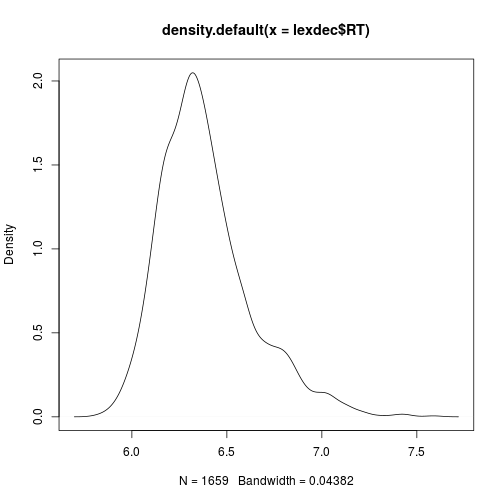lexdec2 <- subset(lexdec, RT < 7) nrow(lexdec2)   1618  lexdec3 <- subset(lexdec2, Correct=="correct") nrow(lexdec3)   1557  ### Inspect subject data to see if there is an effect of Trial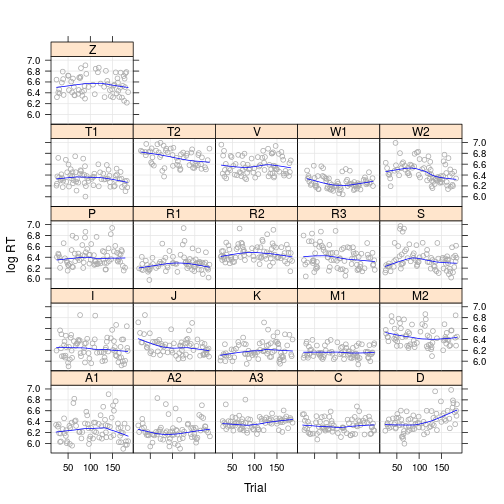### Is it worth including Trial as a random slope within Subject? require(lme4) require(lmerTest) lexdec3$cTrial = lexdec3$Trial - mean(lexdec3$Trial)
summary(m1 <- lmer(RT ~ Frequency + Length + NativeLanguage + cTrial + (1 | Subject) + (1 | Word), data=lexdec3 ))

Linear mixed model fit by REML t-tests use Satterthwaite approximations
to degrees of freedom [lmerMod]
Formula:
RT ~ Frequency + Length + NativeLanguage + cTrial + (1 | Subject) +
(1 | Word)
Data: lexdec3

REML criterion at convergence: -1282.7

Scaled residuals:
Min      1Q  Median      3Q     Max
-2.3184 -0.6624 -0.1181  0.5248  4.4652

Random effects:
Groups   Name        Variance Std.Dev.
Word     (Intercept) 0.002072 0.04552
Subject  (Intercept) 0.014709 0.12128
Residual             0.022569 0.15023
Number of obs: 1557, groups:  Word, 79; Subject, 21

Fixed effects:
Estimate Std. Error         df t value Pr(>|t|)
(Intercept)          6.414e+00  5.472e-02  7.130e+01 117.218  < 2e-16 ***
Frequency           -3.219e-02  5.569e-03  7.320e+01  -5.780 1.71e-07 ***
Length               9.447e-03  3.806e-03  7.200e+01   2.482   0.0154 *
NativeLanguageOther  1.351e-01  5.404e-02  1.900e+01   2.499   0.0218 *
cTrial              -1.841e-04  8.169e-05  1.491e+03  -2.253   0.0244 *
---
Signif. codes:  0 '***' 0.001 '**' 0.01 '*' 0.05 '.' 0.1 ' ' 1

Correlation of Fixed Effects:
(Intr) Frqncy Length NtvLnO
Frequency   -0.660
Length      -0.618  0.427
NtvLnggOthr -0.422 -0.002  0.001
cTrial      -0.002  0.005 -0.003  0.001


### Comparing with a model with Trial as a random effect within Subject

m2 <- lmer(RT ~ Frequency + Length + NativeLanguage + cTrial + (1 + cTrial | Subject) + (1 | Word), data=lexdec3 )
anova(m1, m2)

Data: lexdec3
Models:
object: RT ~ Frequency + Length + NativeLanguage + cTrial + (1 | Subject) +
object:     (1 | Word)
..1: RT ~ Frequency + Length + NativeLanguage + cTrial + (1 + cTrial |
..1:     Subject) + (1 | Word)
Df     AIC     BIC logLik deviance  Chisq Chi Df Pr(>Chisq)
object  8 -1311.2 -1268.4 663.59  -1327.2
..1    10 -1341.8 -1288.3 680.92  -1361.8 34.651      2  2.991e-08 ***
---
Signif. codes:  0 '***' 0.001 '**' 0.01 '*' 0.05 '.' 0.1 ' ' 1


See Baayen (2006, section 7.1 for more detailed analysis)

### Difficulties

Specifying the model, especially selecting the random effect structure can be difficult.

In classical ANOVA/hypothesis testing framework, one specifies the maximally complex model and consult the p-values. The model is often overfitting and lacks robustness. For prediction, a model leaving higher order interactions will typically perform better. But model selection is complicated and Psychologist usually avoid it.

• Barr, D. J., Levy, R., Scheepers, C., and Tily, H. J. (2013). Random effects structure for confirma- tory hypothesis testing: Keep it maximal. Journal of Memory and Language, 68(3):255–278.

• Bates, Douglas, Reinhold Kliegl, Shravan Vasishth, and Harald Baayen. 2015. “Parsimonious Mixed Models.” arXiv Preprint arXiv:1506.04967. http://arxiv.org/abs/1506.04967.

### Books on mixed-effects

• R. H. Baayen (2008) Analyzing linguistic data. A practical introduction to Statistics using R, Cambridge University Press.
• N. W. Galwey (2006) Introduction to Mixed Modelling: Beyond Regression and Analysis of Variance, John Wiley & Sons.
• Andrew Gelman and Jennifer Hill (2007). Data Analysis Using Regression and Multilevel/Hierachical Models. Cambridge University Press.